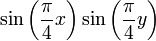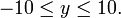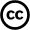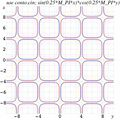# File:ContourPlotExample.jpg

ContourPlotExample.jpg(448 × 448 pixels, file size: 24 KB, MIME type: image/jpeg)

##  Summary

Title / Description: Example of contour plot . Function f(x,y)=$\sin\left(\frac{\pi}{4}x\right) \sin\left(\frac{\pi}{4}y\right)$ is plotted in the range$-10\le x\le 10,$$-10\le y\le 10.$ Levels f(x,y) = 0.1 are shown wiht red lines. Levels f(x,y) = 0.1 are shown wiht blue lines. After to generate the eps file, the image was converted to jpg format.

Author(s): Dmitrii Kouznetsov

Date created: 2008

Country of first publication: Japan

Source: Citizendium

##  LicensingThis work is available under the terms of the Creative Commons Attribution license.

## File history

Click on a date/time to view the file as it appeared at that time.

 Date/Time Thumbnail Dimensions User Comment current 19:47, 22 June 2011448×448 (24 KB) Boris Tsirelson (Title / Description: Example of contour plot . Function f(x,y)= $\sin\left(\frac{\pi}{4}x\right) \sin\left(\frac{\pi}{4}y\right)$ is plotted in the range $-10\le x\le 10,$ $-10\le y\le 10.$ Levels [itex]f(x,y) = − 0.1

The following page links to this file: